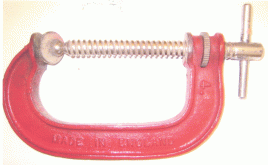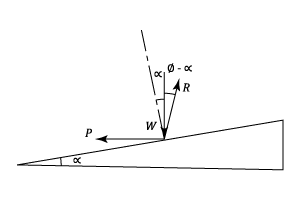I have forgotten

•http://facebook.com/
•https://www.google.com/accounts/o8/id
•https://me.yahoo.com

An analysis of the torque required to turn a screw thread against a known load.View other versions (5)

## Introduction

If the invention of the wheel is an important landmark in the history of civilisation, then the development of the screw thread must run it a close second. Imagine building a car without nuts and bolts. Try to design a machine tool without screw threads to make the adjustments and think of the problems that we would have in factories and workshops without clamps, vices or screw driven lifting appliances like the Screw Jack shown, which is capable of lifting loads of up to 100 tons. Indeed the screw thread in one form or another is truly ubiquitous.

This submission examines the relationships between Torque, Axial Load and Frictional Forces.

## Nut And Screw

A nut is a type of hardware fastener with a threaded hole. Nuts are almost always used opposite a mating bolt to fasten a stack of parts together. The two partners are kept together by a combination of their threads' friction, a slight stretch of the bolt, and compression of the parts. In applications where vibration or rotation may work a nut loose, various locking mechanisms may be employed including adhesives, safety pins or lockwire, nylon inserts, or slightly oval-shaped threads.

A screw thread, often shortened to thread, is a helical structure used to convert between rotational and linear movement or force. A screw thread is a ridge wrapped around a cylinder or cone in the form of a helix, with the former being called a straight thread and the latter called a tapered thread. More screw threads are produced each year than any other machine element.

The development of a screw thread when unwound from the body of a screw is an inclined plane in which the inclination of the plane is equal to the helix angle of the thread.

Strictly speaking the helix angle decreases slightly from the root to the tip of the thread. But the depth of the thread is small compared to the radius of the screw and it is sufficiently accurate to take the helix angle at the mean radius of the thread.

It can be seen from the diagram that:A number of thread forms exist but in this submission only two, the most common, will be considered. In the case ofthreads the included angleis normally. However British Standard Whitworth (B.S.W.), British Standard Fine (B.S.F.), and British Standard Pipe Thread (B.S.T.) use, whilst the small British Association included thread angle is.

The following picture of aclamp uses a square thread.### To Find The Torque Required To Turn A Nut Relative To The Screw Against An Axial Load W:

An angle is the figure formed by two rays sharing a common endpoint, called the vertex of the angle.

A diameter of a circle is any straight line segment that passes through the center of the circle and whose endpoints are on the circle. The diameters are the longest chords of the circle.

•be the mean thread diameter.
•be the Tangential Effort required at the mean thread radius•be the Helix angle of the thread.
•be the angle of friction.

The forces are shown in diagram (b) which represents an equivalent system in which all the load is assumed to be concentrated at one point on the thread. By resolving perpendicular to:The Torque Applied,The Force applied at the end of an arm of lengthFor one turn of the nut (or screw), Input =Or using equation (2), Input =and Output =The efficiency,It can be found by differentiation that the thread angle for maximum efficiency is:If the lead of the screw is,, and since,
Equation (3) can be expanded to giveThe majority of screws are provided withthreads where the normal reaction between the screw and the nut is greater than those found with a square thread. The axial loadshown in the diagram is again assumed to be concentrated at a single point on the thread.

Since the axial component of the Normal Reactionmust be equal to:orWhereis the included angle between the sides of the thread.

But the friction force acts tangentially to the surface of the threads and is given by:Where,and may be regarded as a virtual coefficient of friction.

The conditions for athread, with regards to friction, are identical for those with a square thread provided that the coefficient of frictionis replaced by. i.e. the friction angle is. Once this substitution has been made the equations appertaining to square threads apply.

## (c) Turnbuckle

A turnbuckle, stretching screw or bottlescrew is a device for adjusting the tension or length of ropes, cables, tie rods, and other tensioning systems.

In this a central screw, with right and left hand nuts at each end, turns in nuts at each end. The effect is to double the movement of the load, and double the torque required.

Example:
[imperial]
##### Example - Example 1
Problem
Two parts of a coupling are screwed right and left hand and are jointed by a suitably tapped nut to form a turnbuckle. The threads are square with a lead of and a mean diameter of , the coefficient of friction being .

Find the couple required at the nut to draw the parts together with a force of 3 tons and also the couple necessary to release them.
Workings
The "draw together" phase.

From Equation (3) and toting that the Torque is double for a Turnbuckle:

, the Helix angle of the screw =

The "release" phase

The frictional Force is now reversed and the Forces are now as shown in the diagram.Solution